## Estimation of the static vertical subgrade reaction modulus ks from CPT for flexible shallow foundations on cohesionless soils

 Author(s) Nick Barounis, J Philpot Published 24 November 2017 Collection NZGS Symposium Link N/A Compilation 20th NZGS Symposium
Estimation of the static vertical subgrade reaction modulus ks from CPT for flexible shallow foundations on cohesionless soils

# Abstract

In this paper, an integrated methodology is introduced for estimating the static vertical subgrade reaction modulus from CPT for shallow foundations on cohesionless soils. The proposed methodology relies on fundamental concepts of soil mechanics and foundation engineering applicable to flexible foundations. The methodology returns similar values to the SPT correlation proposed by Scott (Scott, 1981). As a result, it produces values that are in alignment with the traditional SPT approach. The proposed methodology can be used in conjunction with other methods for low risk projects. The methodology is applied to four sites in Christchurch, New Zealand and the results are assessed.

# 1 INTRODUCTION

As an initial step for facilitating the earthquake-resistant design of a building and its foundations, the static spring stiffness, or static vertical subgrade reaction modulus, on the surface of an assumed homogeneous half-space is typically evaluated for the given site. Then, by applying numerous dynamic modification factors to the static spring stiffness (ASCE, 41-13 or Gazetas, 1991), the dynamic spring stiffness can be evaluated. Dynamic modification factors are applied to account for the frequency of the excitation force, the embedment of the foundation and the foundation shape. The most fundamental step in this process for the structural engineer, is to determine if the foundation system under analysis is rigid or flexible. Many references are available for facilitating this step (refer to ASCE 41-13, 2014 or ACI 336). Thus, the estimation of the static spring stiffness is an important step in undertaking both static and earthquake resistant design of foundations. This paper is focused only on the static spring stiffness of flexible foundation systems.

The static vertical subgrade reaction modulus ks is a conceptual relationship, which is defined as the soil pressure exerted σ divided by the deflection δ (Bowles, 1997).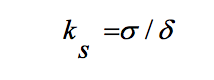(1)

The static vertical subgrade reaction modulus is not an actual engineering property of the soil, such as Poisson’s ratio, as it varies with the width and shape of the foundation (Terzaghi, 1955).

The principle underlying the definition of ks is the resistance a soil layer provides as some deflection is imposed to it due to the applied stress, analogous to a spring shortening at some imposed load. In structural engineering applications, ks is used to model the soil stiffness in the vertical plane when soil-foundation-structure interaction considerations are included in the structural analysis. Typically, as best practice suggests, structural engineers adopt ks values recommended by a geotechnical engineer. The structural engineer further tests the sensitivity of the model for ks values ranging between 0.5 ks and 2.0 ks (ACI 336, 2002). The geotechnical engineer needs to assess the ks range for the particular situation. These values are also to be accompanied by ultimate foundation capacity estimations.

A methodology for the estimation of ks for sands from the Cone Penetration Test (CPT), as an alternative to the conventional correlation to plate load test from the SPT, was proposed by Barounis et al. in 2013. From recent research on the applicability of the method (Barounis and Armaos, 2016), it was demonstrated that the ks values produced by the proposed CPT method are stiffer than the values produced from the conventional SPT method proposed by Scott (Scott, 1981). The stiffer springs from CPT are conservative for the seismic response of the structure, while the softer springs from SPT are conservative for foundation deformation and their effects to the superstructure.

This paper presents an integrated methodology for the estimation of ks values for flexible shallow foundations on cohesionless soils. The methodology produces similar values to the SPT method proposed by Scott for the k1 of a 300mm plate. The final foundation value KF is similar to the value produced by conventional SPT methods.

For a detailed explanation of the background theory to the method, please refer to previous papers from Barounis et al. (2013, 2015, 2016 and 2017) on this topic.

# 2 INTEGRATED METHODOLOGY FOR ESTIMATING THE MODULUS OF SUBGRADE REACTION Ks

## 2.1 Fundamental assumptions, advantages and theoretical basis of the methodology

The fundamental assumptions and theoretical basis for the proposed methodology and its advantages are the following:

• The method is applicable to cohesionless soils, only when tested with a CPT that measures penetration resistance at 10 or 20mm increments with a 35.7mm diameter cone.
• The theory of springs in series is assumed, modified to consider the configuration of soil layers. This means that the equivalent spring stiffness Keq can be estimated by using the proposed methodology.
• The range of SPT N60 values is limited to between 0 and 50 blows. No extrapolation over 50 blows shall be adopted in any case. Thus, an SPT N60 of 50 is considered to be effective refusal.
• This upper bound value of SPT produces a maximum KSPT(0.3) value of 90MN/m3. Thus, any ks values produced using this method for the actual foundation cannot exceed 90MN/m3.
• If values larger than 90MN/m3 need to be adopted, either for the 300mm plate, or for the actual foundation, then actual plate load tests and further site investigations will need to be performed to prove that the subgrade modulus exceeds this value.
• The corresponding qc value of any soil with Ic between 1.00 and 2.60 is related to SPT N60 according to the correlation by Jefferies and Davies, as shown in Figure 8. This figure shows that for increasing Ic values (increasing fines content) at effective SPT refusal, the corresponding qc value reduces from 33.6MPa for Ic=1.00 to 18.7MPa for Ic=2.60. As the fines content increases and for a given N60 value, the cone resistance and the spring stiffness reduces, along with the soil stiffness Es (refer Figure 8).
• The method is applicable for circular, square, continuous or rectangular shallow foundations, founded at any shallow depth in the ground including at the ground surface. The equivalent modulus Keq for any foundation shape is estimated based on the simplified formulae provided by Poulos and Davies (Xiao, 2015) that computes the vertical stress distribution beneath the centre of the foundation.
• By using the Poulos and Davies (Xiao, 2015) formulae, essentially a weighting factor is applied to every 10mm or 20mm long spring. Hence, the soils nearer the foundation become more critical to the overall response than the soils substantially deeper, or outside the pressure bulb of the foundation.
• The method considers an influence depth under any foundation configuration to be the depth at which the vertical stress increase from the foundation becomes equal to 20% of the vertical effective stress (20% rule).
• The method is sensitive to stiffness inversions, i.e. very dense soils overlying very loose soils. The methodology is also sensitive when denser soils are present at some depth from the foundation (within the 20% influence depth). In general, the methodology produces good results, even for highly stratified soils or for sandwiched layers of contrasting stiffness.

The methodology is presented in Table 1. Detailed explanations for each step of the methodology are given in the subsequent paragraphs.

Table 1: Methodology for estimating the spring stiffness of flexible foundations from CPT

 Step # Equation Units Notes 1 For 10mm increment: KCPT=100qc For 20mm increment: KCPT=50qc MN/m3 CPT spring stiffness 2 KCPT (0.3)=0.119KCPT MN/m3 Conversion to 300mm plate spring stiffness 3 KCPT (SPT0.3)= KCPT(0.3) / CF where for 10mm increment: CF=5.67-1.23Ic and for 20mm increment: CF=2.83-0.61Ic MN/m3 Conversion to a similar SPT spring stiffness for a 300mm plate as per Scott’s correlation, KSPT (0.3)=1.8N60 4 MN/m3 Equivalent spring stiffness for a 300mm plate considering an influence depth as per the 20% rule that corresponds to the foundation geometry under analysis 5 KF=Keq x (m+0.5)/(1.5m) where m = L/B MN/m3 Shape correction for the given foundation geometry under analysis

### 2.1.1  1st Step: Estimation of KCPT stiffness

In this first step, the spring stiffness of the 10mm long soil element is estimated. As per equation 1, the spring stiffness is the cone resistance qc divided by the displacement. For a CPT with 35.7mm diameter and a 10mm incremental penetration: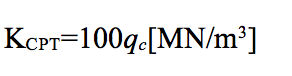(2)

This conversion is applied for every qc measurement until the final CPT depth.

### 2.1.2  2nd Step: Estimation of the 300mm plate by conversion from Step 1

In this step, a conversion takes place from the CPT spring to the equivalent 300mm diameter plate spring. This is undertaken according to the formula proposed by ACI (ACI 336, 2002) and Bowles (Bowles, 1997), which relies on principles earlier presented by Terzaghi (1955):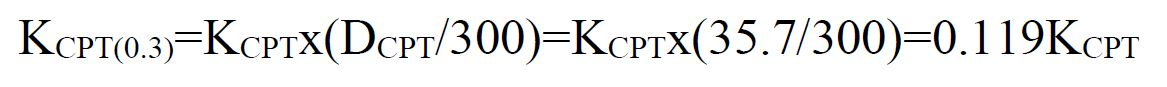(3)

This is consistent with the subgrade reaction modulus theory as a greater loaded area produces lower subgrade reaction values (Terzaghi, 1955 and Bowles, 1997).

### 2.1.3  3rd Step: Conversion to a similar SPT spring stiffness value depending on Ic by means of CF

Jefferies and Davies (1993) have linked the relationship between qc and SPT N60 with the soil behaviour type index Ic. The Ic is defined by Robertson and Wride (Robertson, 2015). The relation is the following: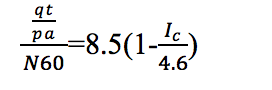(4)

Where qt=qc for cohesionless soils and pa=atmospheric pressure.

By substituting Ic values between 1.00 and 2.60 that correspond to the range of cohesionless soils, pa of 101kPa, and SPT N60 from 0 to 50 as per the assumptions in section 2.1, the relation is depicted in Figure 8.

By dividing the KCPT(0.3) with KSPT(0.3), the stiffness ratio of the CPT spring for the 300mm plate is compared to the SPT spring for the same 300mm plate. We can define this ratio as the Conversion Factor CF: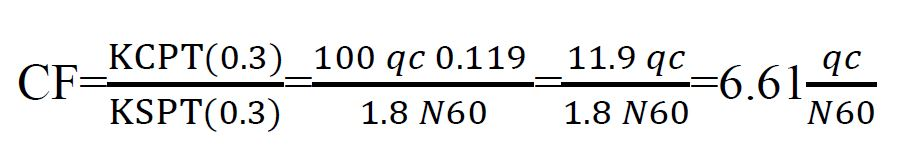(5)

and by solving equation (4) for qc and substituting in (5) we have: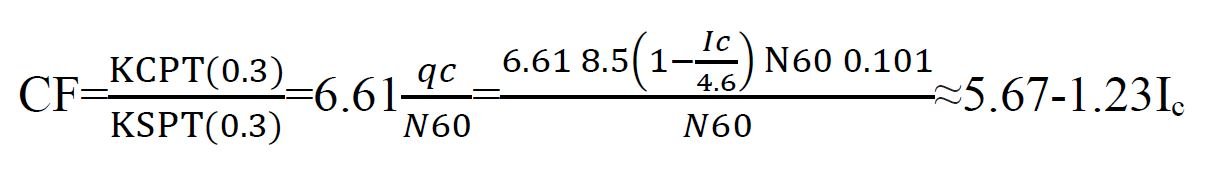(6)

Similarly, for a 20mm increment: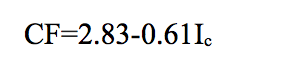(7)

This means that CF is dependent on Ic. If we apply the CF to the KCPT(0.3), then it returns similar spring stiffness for a 300mm plate diameter according to Scott (1981). By using the symbol KCPT(SPT0.3) for the SPT spring stiffness produced by the CPT approach, the equation becomes: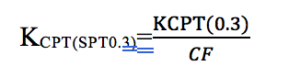(8)

The value of CF can be taken from equations 6 or 7 depending on the increment used.

### 2.1.4  4th Step: Equivalent spring stiffness Keq for a 300mm plate

The general theory of springs suggests that the equivalent spring stiffness Keq of an infinite chain of springs is given by the formula: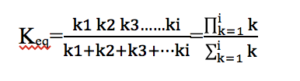(9)

It must be recognised that equation 9 is insensitive to the configuration of soil layers. In other words, the equivalent spring stiffness would return the same value regardless of the sequence with which the soil layers are configured. The assumptions underlying this formula maybe appropriate to be used in structural mechanics; however, it does not accurately capture the soil and foundation behaviour, especially when looser soils are located near the foundation level, or when very dense soils are located at some depth below the foundation, but within the 20% influence bulb. Also, from a numerical perspective, it is impossible for common software to compute the product of 100 individual springs per 1m of CPT. For these reasons, it is proposed to model and capture any layer configuration by using the Boussinesq theory in accordance with the simplified formulae proposed by Poulos and Davies (1974) as presented in Xiao (Xiao, 2015). In essence, the Boussinesq method is used to apply a weighting factor to every KCPT spring within the influence bulb of the foundation. As depth increases, the associated spring stiffness value becomes less significant for the foundation behaviour. Thus, denser deeper soils may not provide substantial stiffness to the foundation, or looser deeper soils may soften the spring substantially. The weighting factor is the well-known influence factor Iz, which takes the value of 1.0 at the foundation depth and diminishes to values that tend to zero with increasing depth.

The proposed formula has the following form: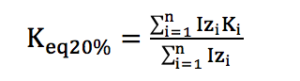(10)

Where Izi is the influence factor that corresponds to a spring stiffness Ki at depth zi as per the following equations (Xiao, 2015):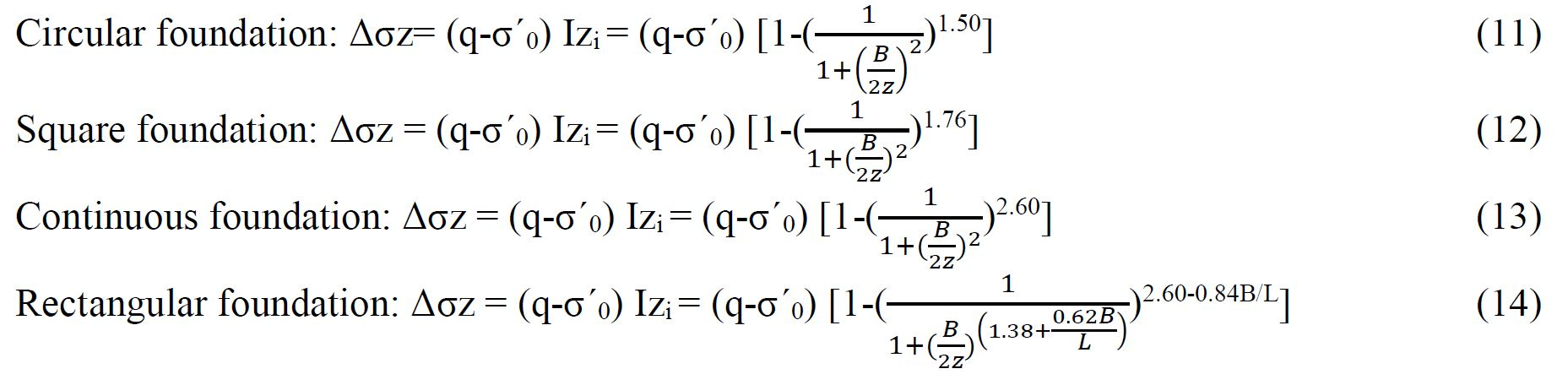where B, L and z are respectively the width, length and depth below the foundation in metres, and (q-σ´0) is the net applied foundation pressure in kPa. Equation 10 is applied to the depth z at which the stress increase from the net applied pressure equals 20% of the vertical effective stress.

### 2.1.5  5th Step: Correction for shape of foundation

In this step, the final foundation spring stiffness value KF is estimated. From the four previous steps, a similar spring stiffness value to the SPT approach has been established. The application of a correction factor for the shape of the foundation with length L and breadth B will also result in a similar corrected spring as for the SPT approach. The shape correction factors are presented in Table 2. The KF is determined from the equation: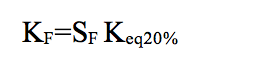(15)

Table 2: Shape correction factors for actual foundation shapes

 Foundation shape Shape correction factor, SF Circular 1.0 Continuous (m+0.5)/(1.5m), m=L/B, tends to 0.67 when L/B≥5 Square 1.0 Rectangular (m+0.5)/(1.5m), m=L/B

# 3 EXAMPLE CALCULATIONS: FOUR SANDY SITES IN CHRISTCHURCH

The proposed methodology has been applied on four different sandy sites in Christchurch, New Zealand. The foundation shape, dimensions, applied pressures and other key information are presented in Table 3.

Table 3: Foundation shape dimensions and applied pressures

 Site Foundation Shape Length (m) Breadth (m) Depth (m) Applied Pressure (kPa) GWT Depth (m) Influence Depth {20% Rule} (m) 1 Rectangular 10 5 0 50 0.6 5.16 2 Continuous 10 2 0 50 1.0 3.02 3 Square 5 5 0 50 1.2 3.44 4 Circular N/A 5 0 50 1.0 3.36

The SBTn plots for all four sites are shown in Figures 1-4 below.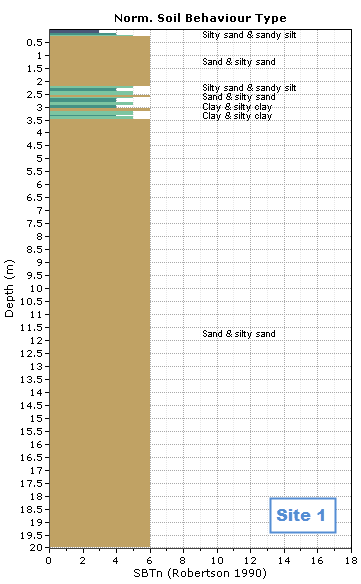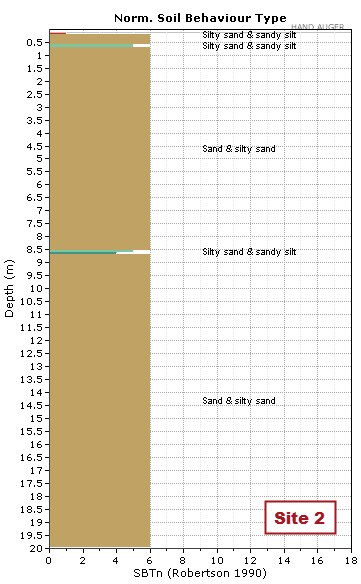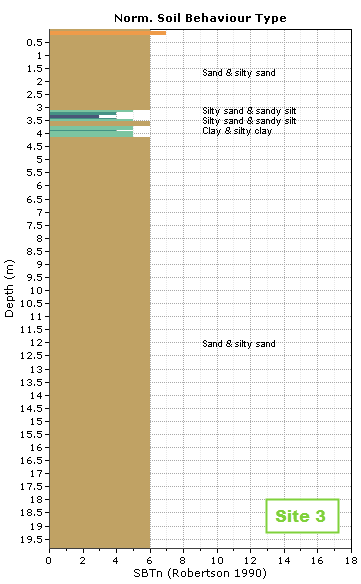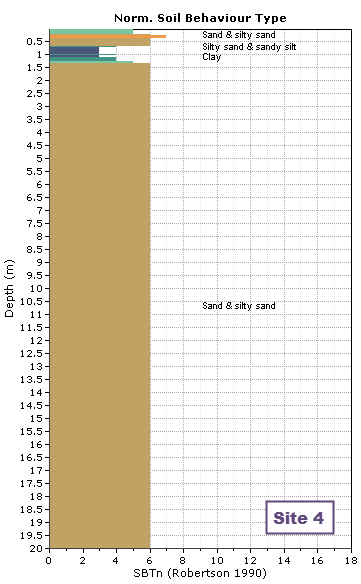Figure 1: SBTn Site 1 – Rectangular Figure 2: SBTn Site 2 – Continuous Figure 3: SBTn Site 3 – Square Figure 4: SBTn Site 4 – Circular

The summary of results from the proposed method is presented in Figures 5-7.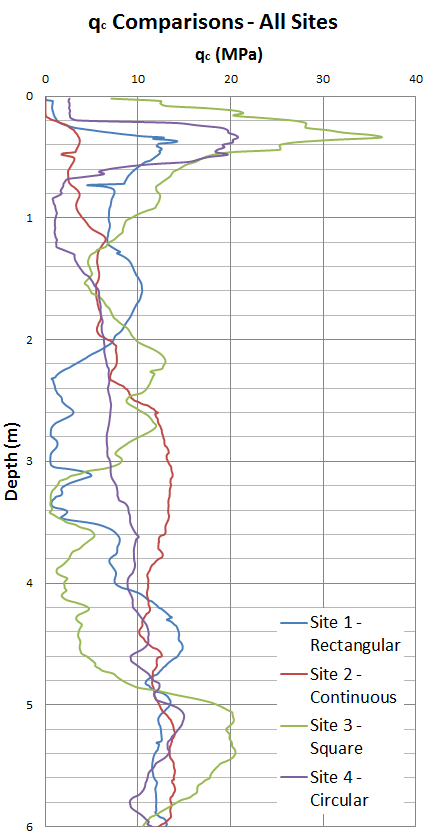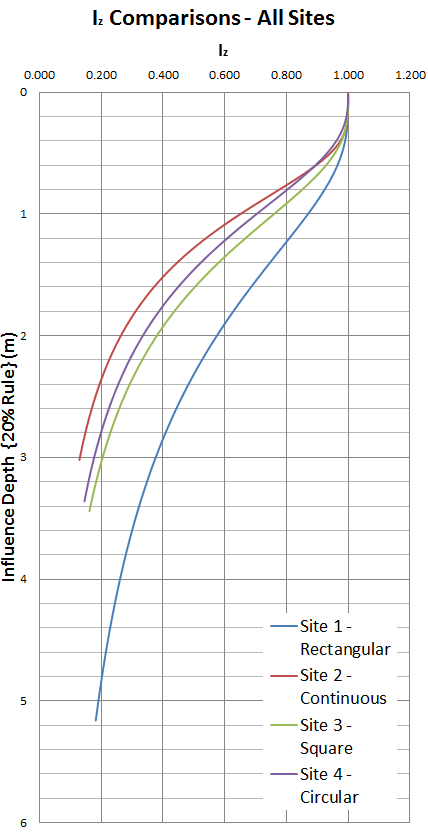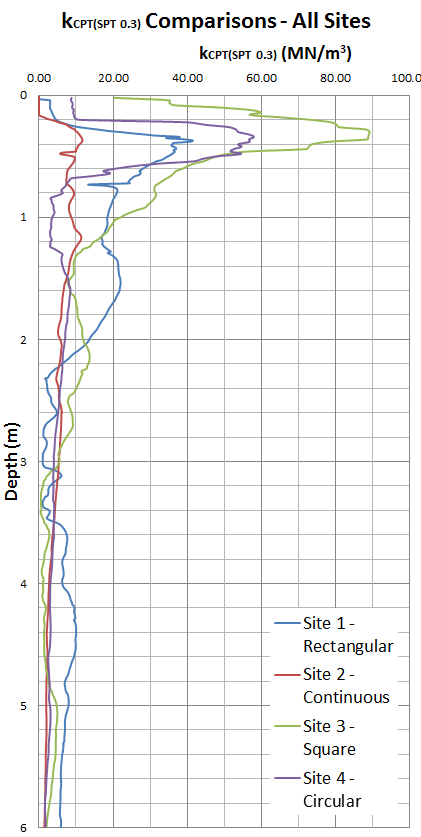Figure 5: Comparison of qc across all four sites Figure 6: Comparison of influence depths (20% rule) across all four sites Figure 7: Comparison of KCPT (SPT 0.3) values across all four sites

The results of the proposed methodology for the four sites and their agreement with Scott’s approach are presented in Table 4. The bar above the N60 and qc values in Table 4 indicates that these are depth weighted values as per Poulos and Davies (1974). These values are equivalent N60 and qc for the influenced soil layers as a whole. These are also plotted in Figure 8 indicating the values are consistent with the soil types encountered at all four sites.

Table 4: Results of the proposed methodologies for four sites in Christchurch

 Site Keq, 20% (MN/m3) KF (MN/m3) 60 c (MPa) Keq, Scott (MN/m3) KF, Scott (MN/m3) 1 21.44 17.86 12 6.84 0.57 21.44 17.87 2 13.60 9.97 8 4.21 0.56 13.60 9.97 3 39.09 39.09 22 13.01 0.60 39.09 39.09 4 21.68 21.68 12 6.89 0.57 21.69 21.69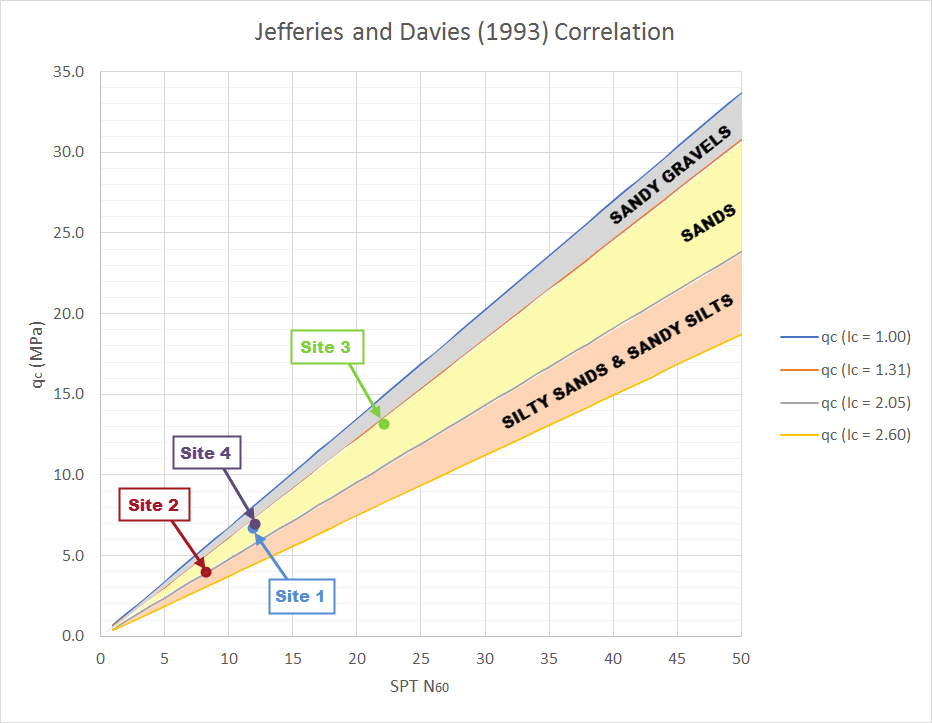Figure 8: Relation between qc and N60 for Ic between 1.00 and 2.60 with depth weighted values plotted for all four sites

# 4 CONCLUSIONS

An integrated method for the estimation of static spring stiffness from CPT has been presented for flexible shallow foundations on cohesionless soils. The method can be applied to estimate the equivalent spring stiffness for a flexible shallow foundation and then subsequently estimate the KF value for the actual foundation size and shape. The method can only be applied for SPT values ranging from 0 to 50, corresponding to a qc that depends on Ic. For facilitating a Winkler foundation type of analysis applicable to flexible foundation systems, ultimate foundation capacity estimations also need to be undertaken by a geotechnical engineer as per well-established available methodologies. The methodology is expected to return similar values, in alignment with the SPT approach as per Scott (1981). However, more research, that includes sensitivity analysis for the proposed methodology, needs to be undertaken to verify the method returns reliable spring values for all possible soil configurations. For assessing KF, one should not rely on one method. A number of methods should be applied, of which this could be one.

# REFERENCES

ACI Committee 336 (2002) Suggested Design Procedures for Combined Footings and Mats. Detroit: American Concrete Institute.

ASCE Standard ASCE/SEI 41-13 (2014) Seismic Evaluation and Retrofit of Existing Buildings. American Society of Civil Engineers.

Barounis, N. and Armaos, P. (2016) Sensitivity analysis of the vertical modulus of subgrade reaction, as estimated from CPT for the design of foundations and for comparison with values from SPT for a site in Christchurch. NZSEE 2016 Conference, Reducing Risk-Raising Resilience, 1-3 April 2016, Christchurch, New Zealand.

Barounis, N. and McMahon, P. (2015) Estimation of vertical subgrade reaction modulus for sands from CPT investigations. 16th European Conference of Soil Mechanics and Geotechnical Engineering, 13-17 September 2015. Edinburgh: UK.

Barounis, N., Saul, G., Lally, D. (2013) Estimation of vertical subgrade reaction coefficient from CPT investigations: applications in Christchurch. Proceedings 19th NZGS Geotechnical Symposium, 2013, Queenstown.

Barounis, N. and Philpot, J. (2017) Estimation of the vertical subgrade reaction modulus ks from CPT for shallow foundations on cohesionless soils. NZSEE 2017 Conference, Next Generation of Low Damage and Resilient Structures, 27-29 April, Wellington, New Zealand.

Bowles, J.E. (1997) Foundation analysis and design, 5th Edition. New York: McGraw-Hill.

Gazetas G. (1991) Formulas and charts for impedances of surface and embedded foundations. Journal of Geotechnical Engineering, ASCE, 113(5), 458-475.

Poulos, H.G and Davis, E.H. (1974) Elastic solutions for soil and rock mechanics. John Wiley, New York.

Robertson, P. K. and Cabal, K.L. (2015) Guide to cone penetration testing, 6th Edition. Gregg Drilling.

Scott, R.F. (1981) Foundation analysis. Prentice Hall, Englewood Cliffs, NJ.

Terzaghi, K. (1955) Evaluation of Coefficients of Subgrade Reaction. Geotechnique, Volume 5, Issue 4, 01 December 1955, pages 297-326.

Xiao, M. (2015) Geotechnical Engineering Design. John Wiley and Sons, Ltd., UK.

Save

Save

Save

SaveNZGS_Symposium_20_Barounis2 (694 KB)